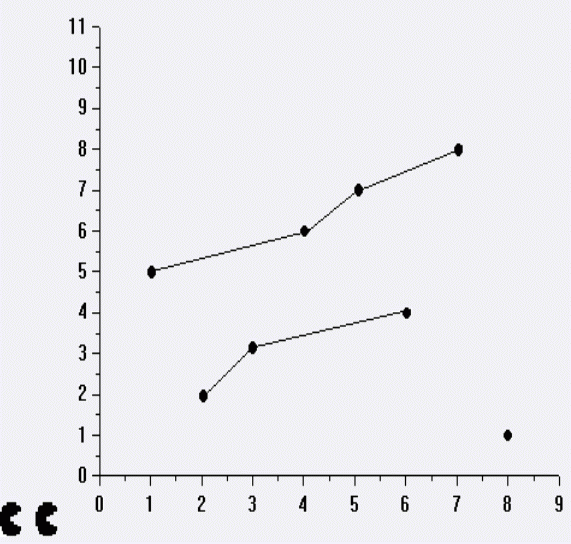# P4066 [SHOI2003]吃豆豆

## 输入输出样例

8
8 1
1 5
5 7
2 2
7 8
4 6
3 3
6 4

### 输出样例#1：

7## 说明：

N < = 2000

Code:

#include <cstdio>
#include <cstring>
#include <algorithm>
#include <queue>
const int N=4050;
const int M=400010;
const int inf=0xcfcfcfcf;
void add(int u,int v,int w,int flow)
{
}
std::pair <int,int > dx[N>>1];
int n;
std::queue <int > q;
int dis[N],used[N],pre[N];
bool spfa()
{
memset(dis,0xcf,sizeof(dis));
dis=0;
q.push(0);
while(!q.empty())
{
int u=q.front();
used[u]=0;
q.pop();
{
int v=to[i],w=edge[i];
if(f[i]&&dis[v]<dis[u]+w)
{
dis[v]=dis[u]+w;
pre[v]=i;
if(!used[v])
{
used[v]=1;
q.push(v);
}
}
}
}
return dis[t]!=inf;
}
void augment()
{
ans+=dis[t];
int now=t;
while(now)
{
f[pre[now]^1]+=1;
f[pre[now]]-=1;
now=to[pre[now]^1];
}
}
int main()
{
scanf("%d",&n);
for(int i=1;i<=n;i++)
scanf("%d%d",&dx[i].first,&dx[i].second);
std::sort(dx+1,dx+1+n);
for(int i=1;i<=n;i++)
{
int mi=-inf;
for(int j=i+1;j<=n;j++)
if(dx[j].second<mi&&dx[j].second>=dx[i].second)
{
mi=dx[j].second;
}
}
t=n*2+2;
for(int i=1;i<=n;i++)
{